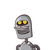# Find the coordinates of the points of equal trisectionof the line segment joining (2,-2)and (-7.4). 2​

Find the coordinates of the points of equal trisection
of the line segment joining (2,-2)and (-7.4). 2​

### 1 thought on “<br /><br />Find the coordinates of the points of equal trisection<br />of the line segment joining (2,-2)and (-7.4). 2​”

1.Given:- A line segment joining the points A(2,−2) and B(−7,4).

Let P and Q be the points on AB such that,

AP=PQ=QB

Therefore,

P and Q divides AB internally in the ratio 1:2 and 2:1 respectively.

As we know that if a point (h,k) divides a line joining the point (x

1

,y

1

) and (x

2

,y

2

) in the ration m:n, then coordinates of the point is given as-

(h,k)=(

m+n

mx

2

+nx

1

,

m+n

my

2

+ny

1

)

Therefore,

Coordinates of P=(

1+2

1×(−7)+2×2

,

1+2

1×4+2×(−2)

)=(−1,0)

Coordinates of Q=(

1+2

2×(−7)+1×2

,

1+2

2×4+1×(−2)

)=(−4,2)

Therefore, the coordinates of the points of trisection of the line segment joining A and B are (−1,0) and (−4,2).